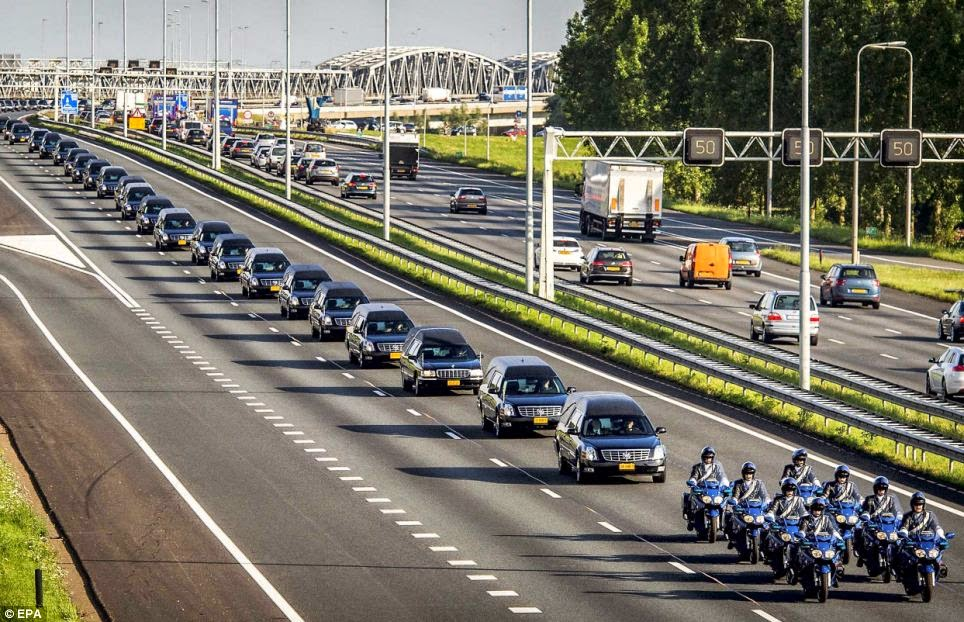## Thursday, July 24, 2014

### 74 Hearse Tribute | The Lengths to Legitimize the MH- 17 Plane Crash Hoax

If you've had a cable television on today you've likely seen the "74 Hearse Tribute" to the downed plane, Flight MH-17, which came on the 18-year anniversary of the TWA800 Flight crash of July 17, 1996.  In the more recent crash, the airline was "MAS", in the seventeen years earlier crash, it was "TWA".
• MAS = 13+1+19 = 33
• TWA = 20+23+1 = 44
• Kill = 11+9+12+12 = 44
What do you know, the two most coded numbers in assassinations and acts of "terrorism" are 33 and 44, and in the case of these airlines, they both have them.  The numbers I refer to as the trinity, are "33, 47 and 74".  They are found paired together in everything from State Names (Oregon) to the Periodic Table, to hurricanes... to more recently, Ukrainian plane crashes.  Ask yourself, what does 74 have to do with anything?  Why do they need a "74 Hearse" tribute?
• Oregon is the 33rd State
• Oregon = 15+18+5+7+15+14 = 74
• Jesus was crucified at 33
• Jesus = 10+5+19+21+19 = 74
• Cross = 3+18+15+19+19 = 74
• Arsenic is the 33rd element on the Periodic Table
• Arsenic = 1+9+1+5+5+9+3 = 33
• Arsenic has an atomic weight of 74
• The qualifying speed for a hurricane?
• If you said 33 meters per second or 74 miles per hour, pat yourself on the back
• That is according to the "Saffir-Simpson" scale
• Saffir = 1+1+6+6+9+9 = 32
• Simpson = 1+9+4+7+1+6+5 = 33If these assholes care so much about Global Warming through CO2 emissions, why is there a 74 Hearse Salute?  Better yet, what happens if you had one part Carbon to Two Parts Oxygen? C+O+O = 3+15+15 = 33